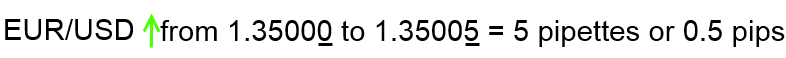Those new to Forex trading may have heard certain terms thrown around a lot without understanding what they mean, here we are going to explain what pips and pipettes are and how they are calculated. It is important that all Forex traders get to grips with pip values before they trade for real.

What Is A Pip?The unit of measurement used to express changes in value between two currencies in a pair is known as a Pip. If the EUR/USD was to rise from 1.3500 to 1.3501, the .0001 rise would be equal to one Pip. Generally a Pip is the last decimal point of a currency quote, with the majority of pairs being quoted out to 4 decimal places. There are however a number of notable exceptions including Japanese Yen pairings which are quoted to two decimal places. So if the USD/JPY, rose from 98.90 to 98.91, this move would equivalent to one pip.

What Is A Pipette or Fractional Pip?You may have noticed that many brokerages quote pairings to 5 and 3 decimal places. These brokerages are quoting fractional pips, also known as pipettes. Using our EUR/USD example a rise from 1.35000 to 1.35005, would be a move of 5 fractional pips or half a full pip. Again if a brokerage was quoting the USD/JPY to three decimal places, a move from 98.900 to 98.905 would be equivalent to 5 fractional pips or half a full pip.

Calculating Pip Value

As you will already know each currency has its own relative value, this makes it necessary to calculate the value of a pip in each particular currency pairing. In the following examples we will be using four decimal point quotes to calculate pip value.

Calculating Pip value is relatively simple and involves the following formula;

.0001 x (1 Unit/ Quote Price) = Pip Value per unit traded

So if the EUR/USD was trading at 1.5200, the calculation would take the following form:

.0001 x (1 / 1.5200) = 0.000152 per unit of currency traded.

So if we were trading 0.01 lots (1,000 notional) each pip would be worth 0.152 Euros (0.000152 * 1000)

If we were trading 0.10 lots (10,000 notional) each pip would be worth 1.52 Euros (0.000152 * 10,000)

If we were trading 1 lot (100,000 notional) each pip would be worth 15.20 Euros (0.000152*100,000)

Calculating Pip Value in your account denomination

The above method demonstrates how you would calculate each the value of each pip in terms of a pairing base currency. But what if your account is held in a different denomination, for instance your account might be held in pounds? Well if that is the case you would be required to calculate pip value in terms in of your account denomination which thankfully isn’t too difficult. To calculate this you simply multiply/divide the pip value by the exchange rate of your account and the currency in question.

Using our EUR/USD example we are going to show you an example of how to calculate Pip value in your account denomination (GBP in this example):

Trading 0.10 lots of the EUR/USD each pip is worth 1.52 Euros, so to calculate this in GBP you would do the following:

1.52 / (1 / .8600) = 1.3072 GBP per pip when trading 0.10 lots.

In the above example you divide instead of multiply as the exchange rate of the two currencies is quoted as EUR/GBP. You would multiply the value, if the value of your denominated account was quoted the other way round. So if the EUR/GBP was in fact quoted as GBP/EUR, you be multiplying instead of dividing in the second half of the above example.

Working out Pip Value

Thankfully you don’t have to go through the slightly laborious process of working out Pip value yourself. There are a number of calculators which will work out pip value for you if you simply input the relevant data. Calculating pip value is important as it can help you better manage your risk, Baby-Pips provide a very simple and easy to use Pip calculator.